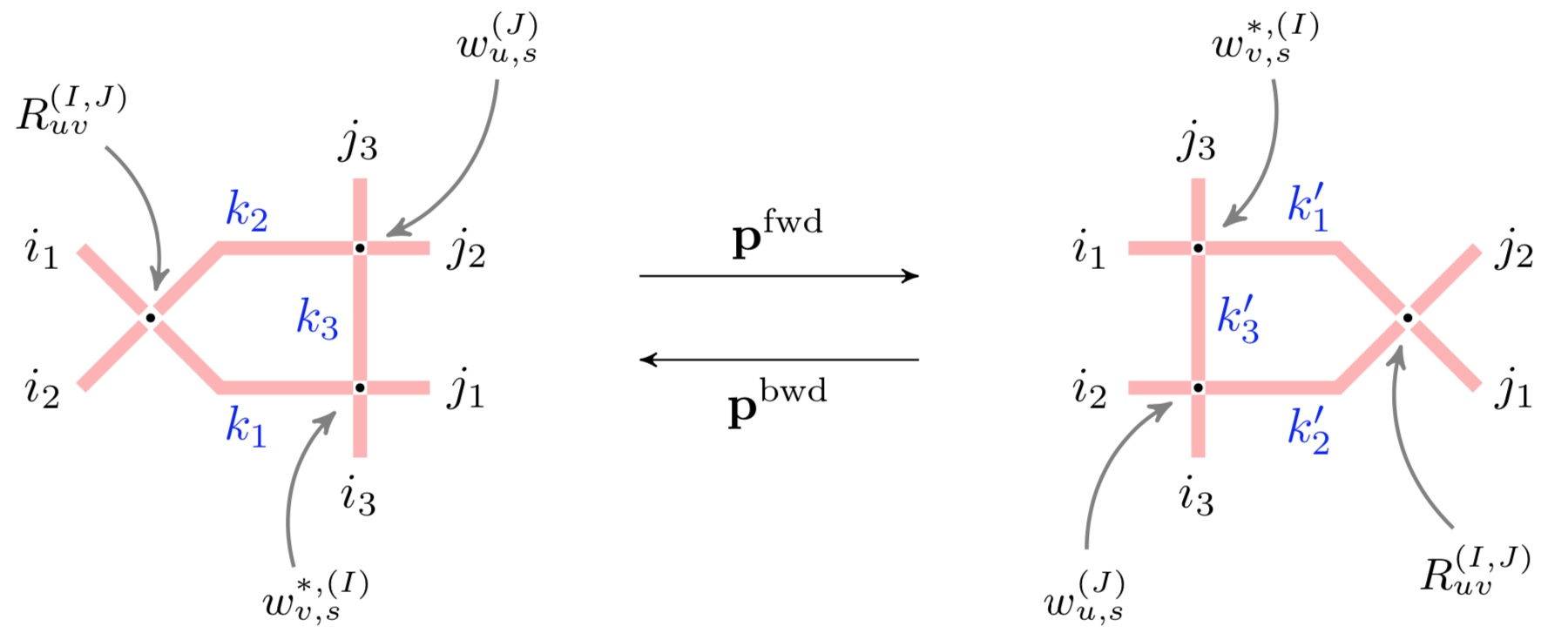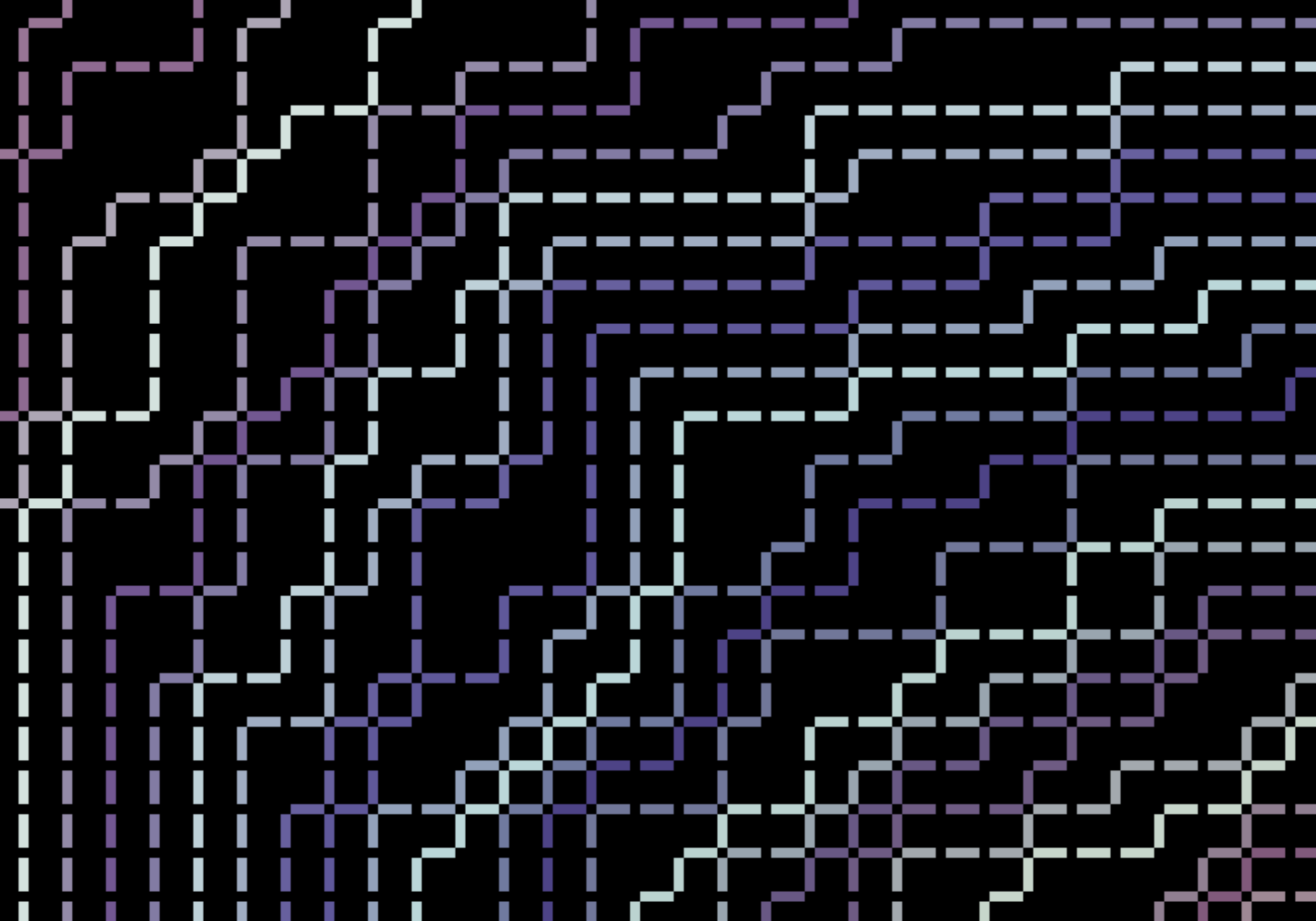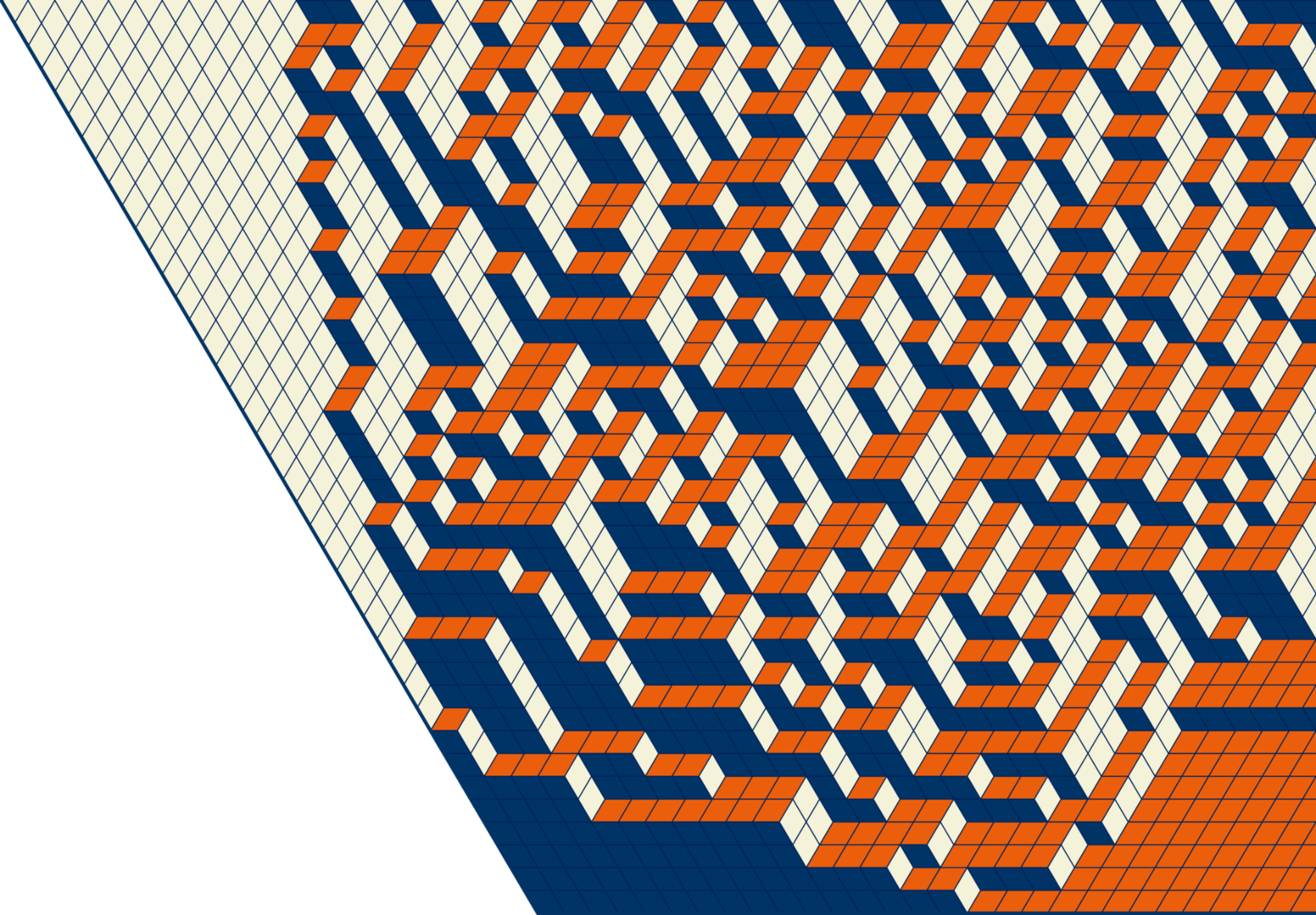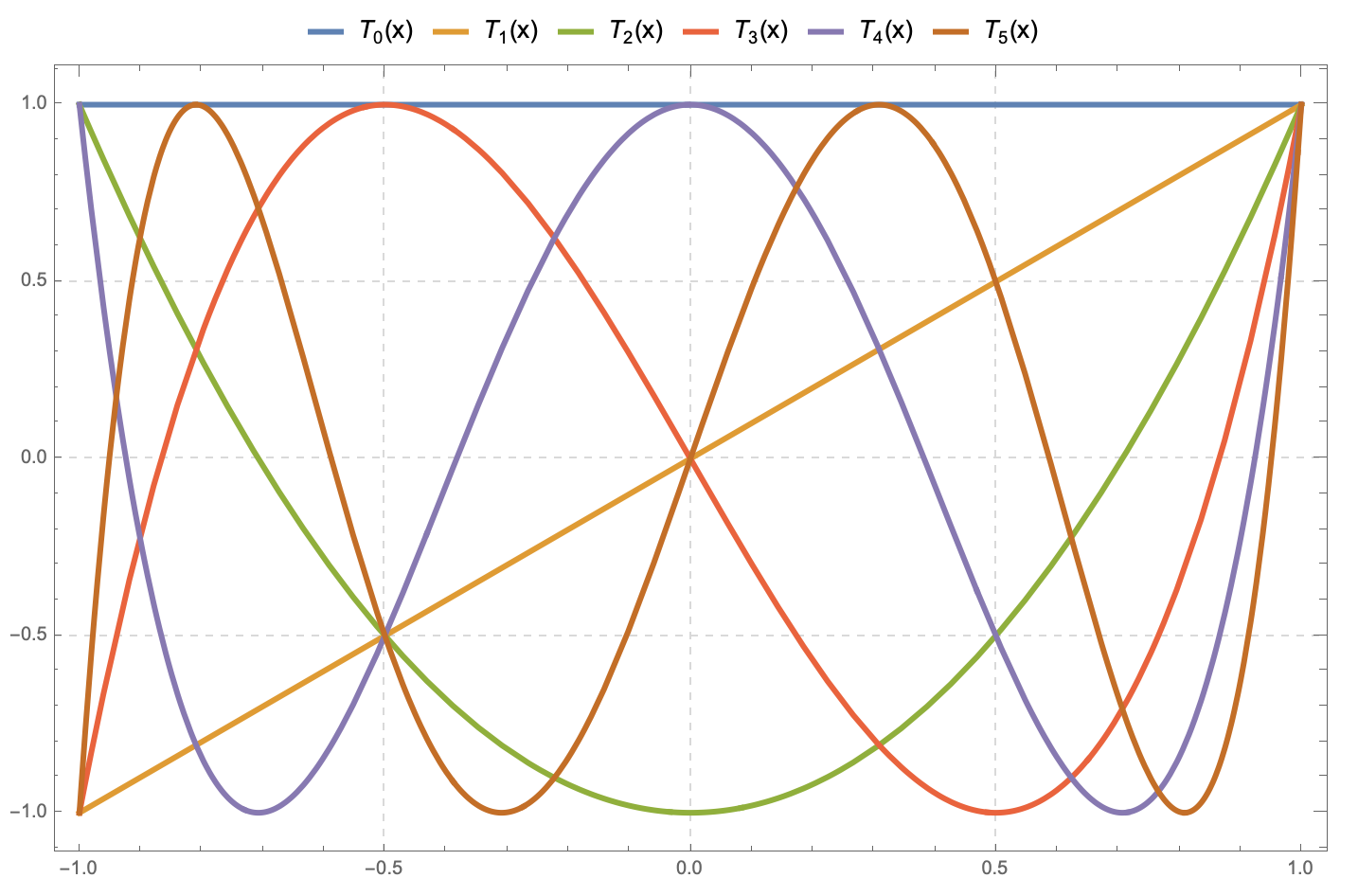### Erratum to "Stochastic higher spin vertex models on the line"

[2019/05/19]

(PDF)

This is an erratum to the paper “Stochastic higher spin vertex models on the line”. The aim of the note is to address two separate errors in the paper: finite vertical spin Plancherel identities, and a false duality claim. The other main statements of the paper (the definition of new stochastic particle systems, duality relations for them, and contour integral observables) are not affected.### Yang-Baxter random fields and stochastic vertex models

[2019/05/15]

Bijectivization refines the Yang-Baxter equation into a pair of local Markov moves which randomly update the configuration of the vertex model. Employing this approach, we introduce new Yang-Baxter random fields of Young diagrams based on spin $q$-Whittaker and spin Hall-Littlewood symmetric functions. We match certain scalar Markovian marginals of these fields with (1) the stochastic six vertex model; (2) the stochastic higher spin six vertex model; and (3) a new vertex model with pushing which generalizes the $q$-Hahn PushTASEP introduced recently by Corwin-Matveev-Petrov (2018). Our matchings include models with two-sided stationary initial data, and we obtain Fredholm determinantal expressions for the $q$-Laplace transforms of the height functions of all these models. Moreover, we also discover difference operators acting diagonally on spin $q$-Whittaker or (stable) spin Hall-Littlewood symmetric functions.### From infinite random matrices over finite fields to square ice

Asymptotic representation theory of symmetric groups is a rich and beautiful subject with deep connections with probability, mathematical physics, and algebraic combinatorics. A one-parameter deformation of this theory related to infinite random matrices over a finite field leads to a randomization of the classical Robinson-Schensted correspondence between words and Young tableaux. Exploring such randomizations we find unexpected applications to six vertex (square ice) type models and traffic systems on a 1-dimensional lattice.### Erratum to "Spectral theory for interacting particle systems solvable by coordinate Bethe ansatz"

[2018/12/19]

(PDF)

This is a correction to Theorems 7.3 and 8.12 in our paper. These statements claimed to deduce the spatial Plancherel formula (spatial biorthogonality) of the ASEP and XXZ eigenfunctions from the corresponding statements for the eigenfunctions of the q-Hahn system. Such a reduction is wrong.### MATH 7310 • Real Analysis and Linear Spaces I

[Spring 2019 semester]### Gibbs measures, arctic curves, and random interfaces

This talk outlines connections between 2-dimensional Gibbs measures with a height function and particle systems in the Kardar-Parisi-Zhang universality class.